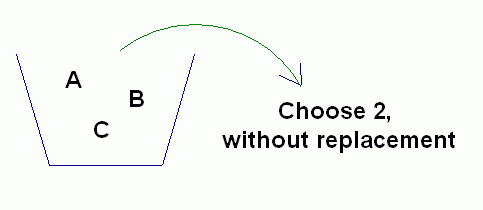﻿ Choosing Things: Does Order Matter?
CHOOSING THINGS: DOES ORDER MATTER?
• PRACTICE (online exercises and printable worksheets)

Many real-life problems involve counting the number of ways that things can be chosen.

In making the choices, sometimes order matters, and sometimes it doesn't.

For example, suppose you're ordering a $3$-topping pizza.
Whether you say ‘Pepperoni, mushrooms, and onions, please’
or ‘Yoo hoo! Make that mushrooms, pepperoni, and onions’
you'll get the same pizza.
Here, order doesn't matter.

Or, suppose you're choosing numbers and letters for a license plate.
The license plate ‘KC 157’ is different from ‘CK 751’,
even though they use the same letters and numbers.
Here, order matters.

LETTERS IN AN URN

Here's another way to think about ‘order matters’ versus ‘order doesn't matter’.

Suppose you have three letters ($\,\text{A}\,$, $\,\text{B}\,$, and $\,\text{C}\,$) in an urn.
You are told to choose two letters, without replacement.
That is, you'll choose a letter, not return it to the urn, and then choose another.
How many ways are there to do this?Well, it depends.
Does order matter, or not?

If order doesn't matter, then there are only three possible choices:
you could walk away with the set $\,\{\text{A},\text{B}\}\,$ or the set $\,\{\text{A},\text{C}\}\,$ or the set $\,\{\text{B},\text{C}\}\,$.

If order does matter, then each of the sets above could be arranged in two different ways,
giving six possible choices:
$\,\text{AB}\ \ \text{BA}\ \ \text{AC}\ \ \text{CA}\ \ \text{BC}\ \ \text{CB}$

PERMUTATIONS versus COMBINATIONS

Mathematicians use two different words, depending on if order matters, or if it doesn't:

DEFINITION permutation (order matters)
A permutation is an arrangement of items in a particular order.

For example, there are six permutations when choosing two letters from $\,\text{A}\,$, $\,\text{B}\,$, and $\,\text{C}\,$:
$$\text{AB}\ \ \ \ \text{BA}\ \ \ \ \text{AC}\ \ \ \ \text{CA}\ \ \ \ \text{BC}\ \ \ \ \text{CB}$$

DEFINITION combination (order doesn't matter)
A combination is a collection of items chosen from a set,
where the order of selection doesn't matter.

For example, there are three combinations when choosing two letters from $\,\text{A}\,$, $\,\text{B}\,$, and $\,\text{C}\,$:
$$\{\text{A},\text{B}\}\ \ \ \ \ \{\text{A},\text{C}\}\ \ \ \ \ \{\text{B},\text{C}\}$$

This author likes to report combinations as sets, to emphasize the fact that order doesn't matter.
(Recall that when using list notation for a set, the order that the members are listed doesn't matter.)

Think of a combination as a bunch of items that are thrown into a basket.
All that matters is what ends up in the basket; it doesn't matter how they got in there.

NOTATION FOR PERMUTATIONS AND COMBINATIONS

there are usually two numbers that are important:

• What is the total number of items that you're choosing from?
This total number is often called $\,n\,$.
In the example above with the letters $\,\text{A}\,$, $\,\text{B}\,$, and $\,\text{C}\,$ in an urn, $\,n=3\,$.
• How many items are you choosing from this total number?
This number (which must be less than or equal to $\,n\,$) is often called $\,k\,$.
When we choose two letters from the urn that contains $\,\text{A}\,$, $\,\text{B}\,$, and $\,\text{C}\,$, then $\,k=2\,$.

NOTATION for PERMUTATIONS and COMBINATIONS
Let $\,n\,$ represent the total number of items that is being chosen from, and let $\,k\le n\,$.

The notation   $\,{}_nC_k\,$   or   $\,\binom{n}{k}\,$   is used to denote the number of ways
to choose $\,k\,$ items from $\,n\,$ (without replacement) when the order doesn't matter.

Thus,   $\,{}_nC_k\,$   or   $\,\binom{n}{k}\,$   denotes the number of combinations that are possible
when choosing $\,k\,$ items from $\,n\,$ items.

Both notations are commonly used.
Both notations may be read aloud as ‘$\,n\,$ choose $\,k\,$’.

The notation   $\,{}_nP_k\,$   is used to denote the number of ways
to choose $\,k\,$ items from $\,n\,$ (without replacement) when the order does matter.

Thus,   $\,{}_nP_k\,$   denotes the number of permutations that are possible
when arranging $\,k\,$ items that are chosen from $\,n\,$ items.

When people are looking at the notation $\,{}_nP_k\,$,
this author reads it aloud by saying three letters in sequence: $\,n\ \ \ P\ \ \ k\,$.

Returning to the experiment of choosing two letters from the urn containing $\,\text{A}\,$, $\,\text{B}\,$, and $\,\text{C}\,$: $${}_3C_2 = \binom{3}{2} = 3\ \ \text{ and }\ \ {}_3P_2 = 6$$ Formulas for both $\,{}_nC_k\,$ and $\,{}_nP_k\,$ will be derived in a future section.
In this web exercise, the emphasis is on understanding the concept of ‘order matters’ versus ‘order doesn't matter’,
and on getting comfortable with the notation.

Master the ideas from this section